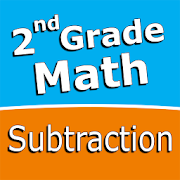# Second grade Math - Subtraction - Lumos Educational App Store31
Price -150
\$50

#### DESCRIPTION:

Intuitive interface powered by handwriting input and a fun and engaging mini game in addition to a regular math trainer mode make our app stand out from the crowd of generic math learning apps. With Second grade Math - Subtraction you can practice and improve the following math skills: - Subtraction facts - numbers up to 20 - Subtract a one-digit number from a two-digit number - Subtract two two-digit numbers - Subtract multiples of 10 - Subtract multiples of 100 - Subtract multiples of 10 or 100 - Subtract three-digit numbers - Balance subtraction equations Choose between two di

#### OVERVIEW:

Second grade Math - Subtraction is a free educational mobile app By Sergey Malugin.It helps students in grades 2 practice the following standards 2.NBT.B.5.

This page not only allows students and teachers download Second grade Math - Subtraction but also find engaging Sample Questions, Videos, Pins, Worksheets, Books related to the following topics.

1. 2.NBT.B.5 : Fluently add and subtract within 100 using strategies based on place value, properties of operations, and/or the relationship between addition and subtraction..

2

#### STANDARDS:

2.NBT.B.5

Developer: Sergey Malugin

Software Version: 5

Category: Education

### RELATED APPSEdSearch WebSearch